Home | | Physics 12th Std | Example Solved Numerical Problems

# Example Solved Numerical Problems

Physics : Semiconductor Electronics: Book Back, Exercise, Example Numerical Question with Answers, Solution: Example Solved Numerical Problems with Answers, Solution

### EXAMPLE 9. 1

An ideal diode and a 5 ╬® resistor are connected in series with a 15 V power supply as shown in figure below. Calculate the current that flows through the diode.### Solution

The diode is forward biased and it is an ideal one. Hence, it acts like a closed switch with no barrier voltage. Therefore, current that flows through the diode can be calculated using OhmŌĆÖs law.

IR

=V/R = 15/5 = 3 A

EXAMPLE 9. 2

Consider an ideal junction diode. Find the value of current flowing through AB is### Solution

The barrier potential of the diode is neglected as it is an ideal diode.

The value of current flowing through AB can be obtained by using OhmŌĆÖs law

V/R = 3 - (-7) / 1├Ś 103 /10= 10 =10-2 = 10mA

### EXAMPLE: 9.3

Find the current through the Zener diode when the load resistance is 1 K╬®. Use diode approximation.### Solution

Voltage across AB is VZ = 9V

Voltage drop across R = 15 - 9 = 6V

Therefore current through the resistor R,

= 6 / 1├Ś10=6 mA

Voltage across the load resistor = VAB = 9V

IL = VAB/RL = 9 / 2├Ś10= 4.5 mA

The current through the Zener diode,

IZ ŌłÆIL =6 mAŌłÆ 4.5mA =1.5 mA

### Optoelectronic devices using Diodes - Numerical Problems Questions with Answers, Solution

EXAMPLE 9.4

Determine the wavelength of light emitted from LED which is made up of GaAsP semiconductor whose forbidden energy gap is 1.875 eV. Mention the colour of the light emitted (Take = 6.6 ├Ś 10-34 Js).

Solution

Eg hc / ╬╗

Therefore

╬╗= hc / Eg  = 6.6├Ś10ŌłÆ34 ├Ś3├Ś108  / 1.875├Ś1.6├Ś10ŌłÆ19

= 660 nm

The wavelength 660 nm corresponds to red colour light.

### EXAMPLE 9. 5

In a transistor connected in the common base configuration, ╬▒=0.95 , IE =1 mA . Calculate the values of IC and IB .

### Solution

╬▒= IC/IE

IC =╬▒ IE =0.95├Ś1=0.95 mA

IE IB IC

Ōł┤ IB IC ŌłÆIE =1ŌłÆ0.95=0.05 mA

### Transistor in Common Emitter Mode - Numerical Problems Questions with Answers, Solution

EXAMPLE 9. 6

The output characteristics of a transistor connected in common emitter mode is shown in the figure. Determine the value of IC when VCE = 15 V. Also determine the value of IC when VCE is changed to 10 V.When VCE = 15 VIC = 1.5 ╬╝A

When VCE is changed to 10 V,  IC = 1.4 ╬╝A

The collector current is independent of the collector- emitter voltage in the active region.

EXAMPLE 9.7

In the circuit shown in the figure, the input voltage Vi is 20 VVBE = 0 and VCE = 0 V. What are the values of IB , IC , ╬▓?### Bipolar Junction Transistor [BJT] - Numerical Problems Questions with Answers, Solution

EXAMPLE: 9.8

The current gain of a common emitter transistor circuit shown in figure is 120. Draw the dc load line and mark the Q point on it. (VBE to be ignored).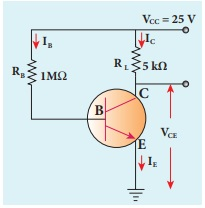Solution

╬▓ = 120### EXAMPLE 9. 9

Calculate the range of the variable capacitor that is to be used in a tuned-collector oscillator which has a fixed inductance of 150 ╬╝H. The frequency band is from 500 kHz to 1500 kHz.### Logic gates - Numerical Problems Questions with Answers, Solution

EXAMPLE 9. 10

What is the output Y in the following circuit, when all the three inputs A, B, and C are first 0 and then 1?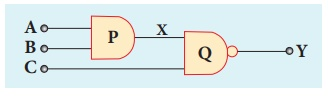### SolutionEXAMPLE 9. 11

In the combination of the following gates, write the Boolean equation for output Y in terms of inputs A and B.### Solution

The output at the 1st AND gate: AThe output at the 2nd AND gate: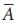The output at the OR gate: AB

De MorganŌĆÖs Theorem - Numerical Problems Questions with Answers, Solution

### EXAMPLE: 9 . 12

Simplify the Boolean identity

AC + ABC = AC

### Solution

Step 1: AC (1 + B) = AC.1 [OR law-2]

Step 2: AC . 1 = AC [AND law ŌĆō 2]

Therefore, AC + ABC = AC

Circuit DescriptionThus the given statement is proved.

### EXAMPLE 10.1

A transmitting antenna has a height of 40 m and the height of the receiving antenna is 30 m. What is the maximum distance between them for line-of-sight communication? The radius of the earth is 6.4├Ś106 m.### Solution:

The total distance between the transmitting and receiving antennas will be the sum of the individual distances of coverage.

d1 +d2

= ŌłÜ(2Rh┬Ł) + ŌłÜ(2Rh2)

= ŌłÜ2ŌłÜ(ŌłÜh1 + ŌłÜh2)

= ŌłÜ[2 ├Ś 6.4 ├Ś106] ├Ś ( ŌłÜ40 + ŌłÜ30 )

=16 ├Ś102 ŌłÜ5 ├Ś(6.32 + 5.48)

=42217m= 42.217 km

Numerical Problems

1. The given circuit has two ideal diodes connected as shown in figure below. Calculate the current flowing through the resistance R1 [Ans: 2.5 A]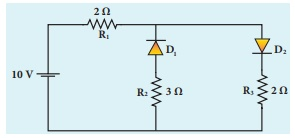Solution:

Barrier potential for ideal diode is zero. The diode D1 is reverse biased, so it will block the current and diode Dis forward biased, so it will pass the current.

The given circuit becomesEffective resistance Reff = R1 + R3 = 4╬®

Current through R1 = V/Reff = 10/4 = 2.5A

2. Four silicon diodes and a 10 ╬® resistor are connected as shown in figure below. Each diode has a resistance of 1╬®. Find the current flows through the 18╬® resistor. [Ans: 0.13 A]Solution:

In the given circuit D2 & D3 are in forward bias so they conduct current while D1 &D4 are in reverse bias so they do not conduct current. So the equivalents circuit will be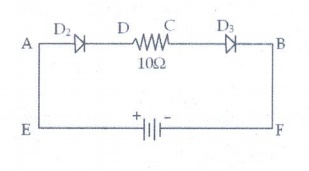the effective resistance is Reff

= 1╬® + 10╬® + 1╬® = 12╬®

Here silicon diodes are used

Ōł┤ Barrier potential for Si is 0.7 V

Net potential (Vnet) = 3 ŌĆō 0.7 - 0.7

Vnet = 1.6 V

Current (I) = Vnet / R eff = 1.6 / 12 = 0.133A

3. Assuming VCEsat = 0.2 V and ╬▓ = 50, find the minimum base current (IB) required to drive the transistor given in the figure to saturation. [Ans: 56 ┬ĄA]Solution;

VCE = 0.2V

Rc = 1k╬®

╬▓ =50

Vcc = 3V

I B = ?Ic = [ VCC ŌĆō VCE ] / RC

= (3-0.2) / 103

= 2.8 ├Ś 10-3 A

= 2.8 mA

╬▓= IC/IB

Ōł┤ IB = IC / ╬▓ = 2.8 ├Ś 10-3 / 50 = 0.056├Ś 10-3

= 56 ├Ś 10-6 A

IB=56╬╝A

4. A transistor having ╬▒ =0.99 and VBE = 0.7V, is given in the circuit. Find the value of the collector current.

[Ans: 5.33 mA]Given data:

╬▒ =0.99

VBE = 0.7V

IC=?

Solution:Tranistor is in saturation region.

Ōł┤ Ic = Ic(sat)

IC and IB are independent

VCE(sat) = 0.2 V, VBE(sat) = 0.8 V for silicon transistor (ie, standard value)

Apply KVR across B-E loop:

V1k + V10k + VBE sat + V1k = VCC

Ōł┤ 1(IC + IB) + 10 IB + 0.8 + 1 (IC + IB) = 12

2 IC + 12 IB = 11.2         ŌĆ”ŌĆ”ŌĆ”ŌĆ”ŌĆ”(1)

Apply KVR across C-E loop:

V1k + V1k + VCE sat + V1k = VCC

l(IC + IB) + 1 IC + 0.2 + I(IC + IB) = 12

3IC + 2IB = 11.8    ŌĆ”ŌĆ”ŌĆ”ŌĆ”ŌĆ”ŌĆ”.(2)

Solve the equation (1) and (2)

IB = 0.3125 mA

IC = 3.725 mA Ōēł 3.73 mA

5. In the circuit shown in the figure, the BJT has a current gain (╬▓) of 50. For an emitter ŌĆō base voltage VEB = 600 mV, calculate the emitter ŌĆō collector voltage VEC (in volts). [Ans: 2 V]Given data:

╬▓ = 50

VE=3V

VEB=60 mV

RB=60K╬®

RE=500╬®

Solution:

VB=VEŌłÆVEB

VB = 3ŌłÆ0.6 = 2.4V

I= VB/RB = 2.4 / 60├Ś103 = 40╬╝A

I= ╬▓IB = 50├Ś40╬╝A = 2├Ś10-3 A = 2mA

VC = RCIC = 500├Ś2├Ś10-3 = 1V

VEC = VE-V= 3-1 = 2V

VEC = 2V

6. Determine the current flowing through 3╬® and 4╬® resistors of the circuit given below. Assume that diodes D1 and D2 are ideal diodes.Solution:

The diode D2 is in reverse biased. So does not conduct current.

Ōł┤ current through 3╬® is = 0

The diode Dis in forward biased, and it is an ideal diode. So the given circuit becomes asThe current through 4╬® is

Ōł┤ I = V/R = 12/6 =2A

7. Prove the following Boolean expressions using the laws and theorems of Boolean algebra.

i) (A + B) (A +) = A

ii) A  (+B) = AB

iii) (A + B) (A + C) = A + BC

Solution:8. Verify the given Boolean equation A +  ßŠ╣B = A + B using truth table.

Solution:Hence, verified

9. In the given figure of a voltage regulator, a Zener diode of breakdown voltage 15V is employed. Determine the current through the load resistance, the total current and the current through the diode. Use diode approximation.Solution:

Voltage across RL(VO) = Vz = 15V

Voltage across RS(VRS) = 25 -15 = 10V

current through RL is

IL = V0/RL = 15 / 3├Ś103 = 5├Ś10-3 A

I= 5 mA

Current through RS is

I = VRS/RS = 10/500 = 20├Ś10-3A

I = 20mA

Current through Zener diode is

IZ=I-IL

= (20-5) ├Ś 10-3

Iz = 15mA

10. Write down Boolean equation for the output Y of the given circuit and give its truth table.Solution:Tags : with Answers, Solution | Semiconductor Electronics | Physics , 12th Physics : UNIT 10a : Semiconductor Electronics
Study Material, Lecturing Notes, Assignment, Reference, Wiki description explanation, brief detail
12th Physics : UNIT 10a : Semiconductor Electronics : Example Solved Numerical Problems | with Answers, Solution | Semiconductor Electronics | Physics